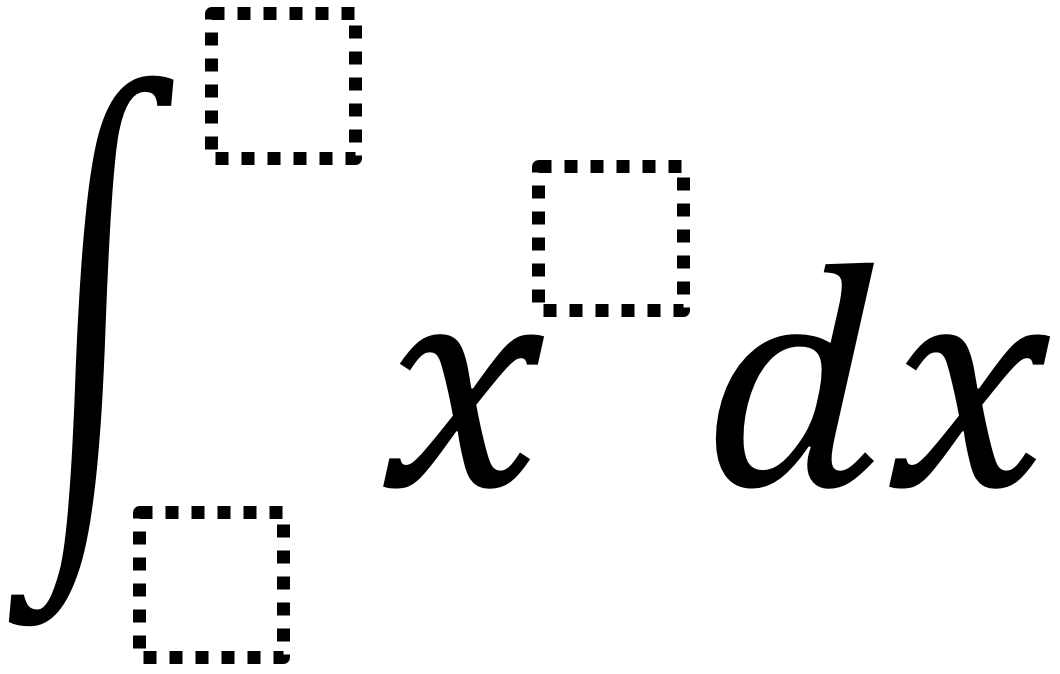Home > Calculus > Definite Integral 3

# Definite Integral 3

Directions: Use the digits 1 to 9, at most one time each, to fill in the boxes and make a solution that is as close to 100 as possible.### Hint

How do the upper and lower bounds determine whether the solution is negative or positive?

I only know how to figure this out via brute force and would love to learn if anyone has a conceptual approach to this.

I created this spreadsheet and tried all 9 potential exponent values. The bounds and exponent that result in a value as close to 100 as possible are:

upper bound: 8
lower bound: 6
exponent: 2
value: 98.666…

and a close second place is:

upper bound: 2
lower bound: 1
exponent: 9
value: 102.30

Source: Robert Kaplinsky

## Derivative of Trig Functions 2

Directions: Fill in the boxes below using the digits 1 to 6, at most one …

1.I created a desmos graph to quickly sift through solutions. I integrated leaving the exponent as a variable, and then used “x” and “y” to be the upper and lower bound and set the whole thing equal to 100. I used the slider to change the exponent “c”, and then found solutions for x and y where x is not equal to y and the points are approximately integral. I came up with exponent 2, upper bound 8, lower bound 6; exponent 3, upper bound 5, lower bound 4; and exponent 9, upper bound 2, lower bound 1. The optimal solution is exponent 2, which is 98.6666666…

Here is the desmos graph I used: https://goo.gl/QEc25d

•This is really cool Alison! I had never thought about it visually. I’ll need to think about this more.

2.One of my students did the integral from 0 to 2.9 for x^5. She wondered if she could use it since the problem didn’t restrict them to integers. 😉

•I guess that could work, but by “digit” it was intended to be an integer. Creative thinking though!